One by One [ 1 x 1 ] Convolution - counter-intuitively useful

Whenever I discuss or show GoogleNet architecture, one question always comes up -

"Why 1x1 convolution ? Is it not redundant ?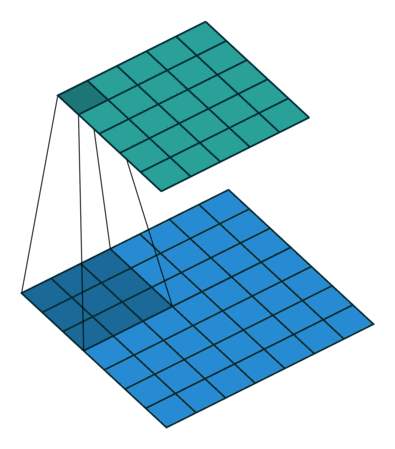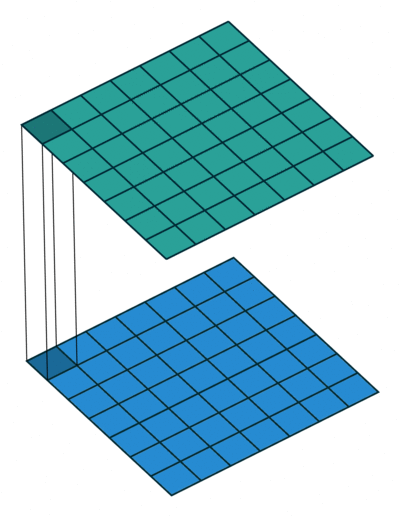left : **Convolution with kernel of size 3x3** right : **Convolution with kernel of size 1x1**

Most simplistic explanation would be that 1x1 convolution leads to dimension reductionality. For example, an image of 200 x 200 with 50 features on convolution with 20 filters of 1x1 would result in size of 200 x 200 x 20. But then again, is this is the best way to do dimensionality reduction in the convoluational neural network? What about the efficacy vs efficiency?

Feature transformation

Although 1x1 convolution is a ‘feature pooling’ technique, there is more to it than just sum pooling of features across various channels/feature-maps of a given layer. 1x1 convolution acts like coordinate-dependent transformation in the filter space. It is important to note here that this transformation is strictly linear, but in most of application of 1x1 convolution, it is succeeded by a non-linear activation layer like ReLU. This transformation is learned through the (stochastic) gradient descent. But an important distinction is that it suffers with less over-fitting due to smaller kernel size (1x1).

Deeper Network

One by One convolution was first introduced in this paper titled Network in Network. In this paper, the author’s goal was to generate a deeper network without simply stacking more layers. It replaces few filters with a smaller perceptron layer with mixture of 1x1 and 3x3 convolutions. In a way, it can be seen as “going wide” instead of “deep”, but it should be noted that in machine learning terminology, ‘going wide’ is often meant as adding more data to the training. Combination of 1x1 (x F) convolution is mathematically equivalent to a multi-layer perceptron..

Inception Module

In GoogLeNet architecture, 1x1 convolution is used for two purposes

• To make network deep by adding an “inception module” like Network in Network paper, as described above.
• To reduce the dimensions inside this “inception module”.
• To add more non-linearity by having ReLU immediately after every 1x1 convolution.

Here is the scresnshot from the paper, which elucidates above points :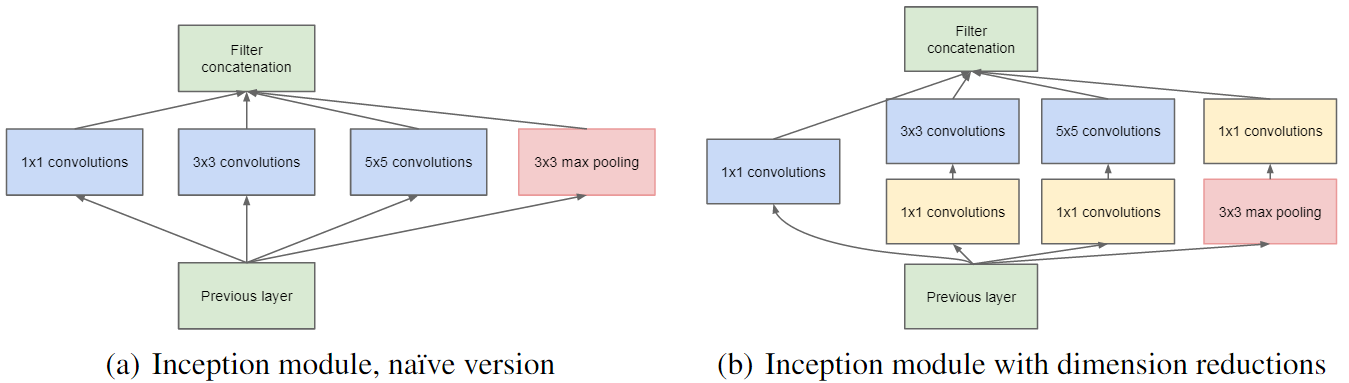It can be seen from the image on the right, that 1x1 convolutions (in yellow), are specially used before 3x3 and 5x5 convolution to reduce the dimensions. It should be noted that a two step convolution operation can always to combined into one, but in this case and in most other deep learning networks, convolutions are followed by non-linear activation and hence convolutions are no longer linear operators and cannot be combined.

In designing such a network, it is important to note that initial convolution kernel should be of size larger than 1x1 to have a receptive field capable of capturing locally spatial information. According to the NIN paper, 1x1 convolution is equivalent to cross-channel parametric pooling layer. From the paper - “This cascaded cross channel parameteric pooling structure allows complex and learnable interactions of cross channel information”.

Cross channel information learning (cascaded 1x1 convolution) is biologically inspired because human visual cortex have receptive fields (kernels) tuned to different orientation. For e.gDifferent orientation tuned receptive field profiles in the human visual cortex Source

More Uses

• 1x1 Convolution can be combined with Max pooling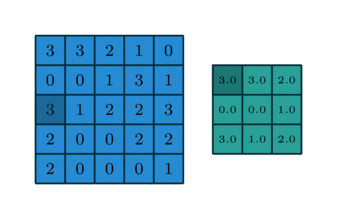Pooling with 1x1 convolution

• 1x1 Convolution with higher strides leads to even more redution in data by decreasing resolution, while losing very little non-spatially correlated information.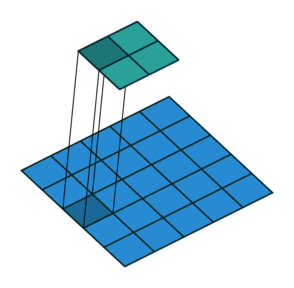1x1 convolution with strides

• Replace fully connected layers with 1x1 convolutions as Yann LeCun believes they are the same -

In Convolutional Nets, there is no such thing as “fully-connected layers”. There are only convolution layers with 1x1 convolution kernels and a full connection table. – Yann LeCun

Convolution gif images generated using this wonderful code, more images on 1x1 convolutions and 3x3 convolutions can be found here

Written on March 25, 2016